# ishi

Graph plotting package with a small API and sensible defaults powered by gnuplot
0.7.2 Latest release released

# 石の上にも三年

Graph plotting package with a small API and sensible defaults powered by gnuplot.

Requires gnuplot.

## Installation

1. Add the dependency to your `shard.yml`:
``````dependencies:
ishi:
github: toddsundsted/ishi
``````
1. Run `shards install`

## Usage

To display a line chart of the data points in `xdata` (the x values) and `ydata` (the corresponding y values):

``````require "ishi"

ishi = Ishi.new
ishi.plot(xdata, ydata)
ishi.show
``````

Or, if you prefer command-style syntax:

``````require "ishi"

Ishi.new do
plot(xdata, ydata)
end
``````

A chart can display multiple plots. The following code displays two plots in one chart: one derived from discrete data points and the other from the equation of a line.

``````require "ishi"

Ishi.new do
plot([1, 2, 3, 4, 5], [1.0, 1.4, 1.9, 2.4, 2.6], "ko", title: "data")
plot("0.4 * x + 0.7", "b--")
end
``````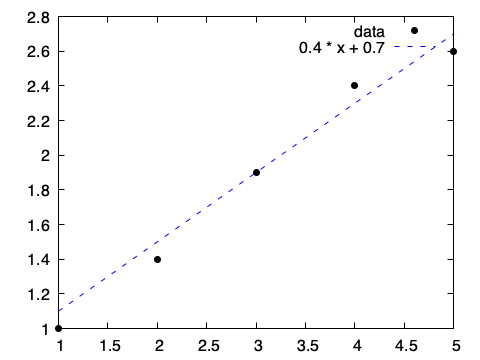The etc/examples directory contains examples of usage.

### plot

`plot` plots points and lines. It takes data in several formats:

• `plot(ydata)` - y values in ydata with x values ranging from `0` to `ydata.size - 1`
• `plot(xdata, ydata)` - x values in xdata and corresponding y values in ydata
• `plot(xdata, ydata, zdata)` - x values in xdata, y values in ydata, and z values in zdata
• `plot(expression)` - any gnuplot-supported mathematical expression

xdata, ydata and zdata may be any type that implements `Indexable(Number)`. Chart dimensionality (2D or 3D) is inferred from the data.

All `plot` methods/commands accept the optional named arguments title, style, dashtype (dt), linecolor (lc), linewidth (lw), pointsize (ps), pointtype (pt), linestyle (ls), fillstyle (fs) and format.

title specifies the title of the plot in the chart key.

style explicitly specifies the style of the plot (`:lines`, `:points`, etc.). Ishi will try to infer the style from the data and the other named arguments (for example, if both linewidth and pointsize are provided, the style will be `:linespoints`).

By default, plots are rendered with solid lines. dashtype (dt) specifies the pattern of dashes to use instead. dashtype may be an array of pairs of numbers that specify the length of a solid line followed by the length of an empty space (e.g. `[2, 3, 5, 7]`), or it may be a string composed of . (dot), - (hyphen), _ (underscore) and (space), which is then converted into an array as follows: each "." becomes `[2, 5]`, "-" becomes `[10, 10]`, "_" becomes `[20, 10]` and " " adds 10 to the previous empty value.

linecolor specifies the color to use for lines and points. Colors may be specified by name (e.g. "red", "blue", or "green") or by hexadecimal color value (e.g. "#AARRGGBB" or "#RRGGBB").

linewidth and pointsize scale the width of lines and the size of points, respectively. A value of 2.0 is twice as big as the default width or size.

The following code demonstrates the use of dashtype, linecolor and linewidth:

``````require "ishi"

Ishi.new do
plot("x + 4.0", dashtype: "-", linewidth: 2)
plot("x + 3.0", dashtype: "_", linewidth: 2)
plot("x + 2.0", dashtype: ".", linewidth: 2)
plot("x + 1.0", dashtype: "..._", linewidth: 2)
plot("x + 0.0", dashtype: [30, 10, 50, 10], linewidth: 2, linecolor: "#88001100")
end
``````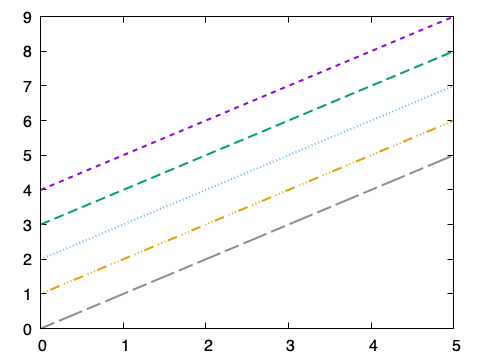pointtype selects the type of point to render. Available types depend on the gnuplot terminal device used, but commonly supported values are . (dot), + (plus sign), x (multiplication sign), * (asterisk), s (square), o (circle), ^ (up triangle), and v (down triangle) and d (diamond).

The following code demonstrates the use of pointtype and pointsize:

``````require "ishi"

Ishi.new do
plot([1, 2, 3, 4], [5, 6, 7, 8], pointtype: 1, pointsize: 2)
plot([1, 2, 3, 4], [4, 5, 6, 7], pointtype: "o", pointsize: 2)
plot([1, 2, 3, 4], [3, 4, 5, 6], pointtype: "s", pointsize: 2)
plot([1, 2, 3, 4], [2, 3, 4, 5], pointtype: "^", pointsize: 2)
plot([1, 2, 3, 4], [1, 2, 3, 4], pointtype: "v", pointsize: 2, linecolor: "#88001100")
end
``````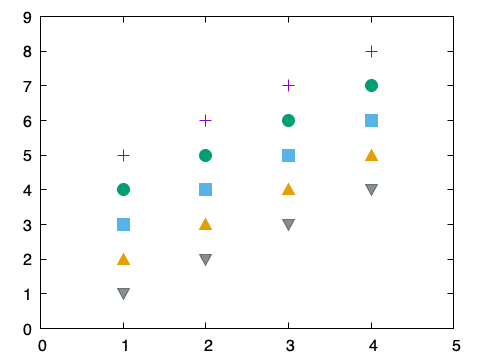The format argument is a short string used to specify color, point and line, simultaneously.

Color is a letter from the set b (blue), g (green), r (red), c (cyan), m (magenta), y (yellow), k (black) or w (white). Point is a letter from the set . (dot), + (plus sign), x (multiplication sign), * (asterisk), s (square), c (circle), ^ (up triangle), v (down triangle) or d (diamond). Line may be - (solid line), -- (dashed line), ! (dash-dot line) and : (dotted line).

Given these rules, the format string "b" is a solid blue line, "or" is red circles, "^k:" is black triangles connected by dotted black lines, "g-" is a solid green line and "--" is a dashed line.

format may also be a single word color name or hexadecimal color value, in which case point and line may not be specified.

Returning to the first example, the code uses format to customize the plots:

``````require "ishi"

Ishi.new do
plot([1, 2, 3, 4, 5], [1.0, 1.4, 1.9, 2.4, 2.6], "ko", title: "data")
plot("0.4 * x + 0.7", "b--")
end
``````

The format "ko" could also be expressed explicitly (and much more verbosely) with the named arguments `linecolor: "black", pointtype: 7`, and the format "b--" with `linecolor: "blue", dashtype: 2`.

### scatter

`scatter` draws scatter plots. It takes data in several formats:

• `scatter(xdata, ydata)` - x values in xdata and corresponding y values in ydata
• `scatter(xdata, ydata, zdata)` - x values in xdata, y values in ydata, and z values in zdata

Chart dimensionality (2D or 3D) is inferred from the data. By default, `scatter` places a . (dot) at each point.

All `scatter` methods/commands accept the optional named arguments title, style, dashtype (dt), linecolor (lc), linewidth (lw), pointsize (ps), pointtype (pt), linestyle (ls) and format.

The following code demonstrates the use of scatter:

``````require "ishi"

Ishi.new do
scatter(xdata, zdata, lc: "#4b03a1")
scatter(ydata, zdata, lc: "#b5367a")
end
``````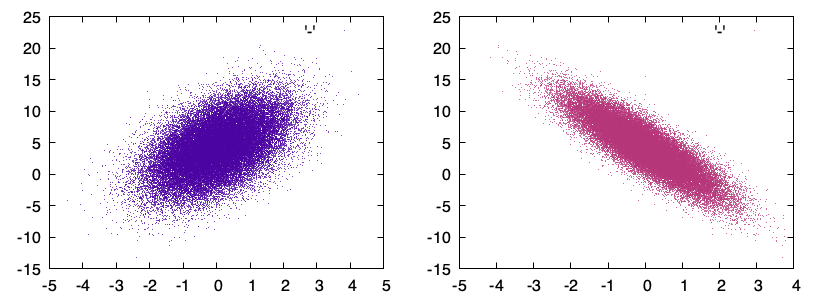### imshow

`imshow` displays data as a pseudocolor, heatmapped image:

• `imshow(data)` - data is two-dimensional scalar data, which will be rendered as an image

The following code demonstrates the use of imshow:

``````require "ishi"

Ishi.new do
palette(:inferno)
imshow(data)
margin(0, 0, 0, 0)
show_colorbox(false)
show_border(false)
show_xtics(false)
show_ytics(false)
show_key(false)
end
``````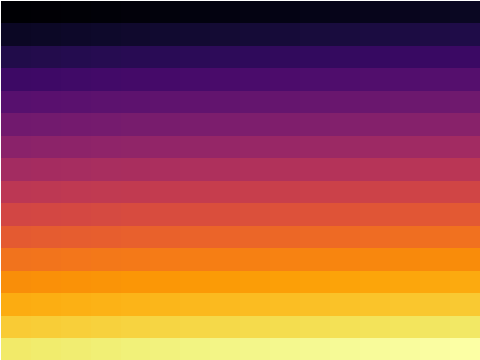### charts

`charts` changes the number of charts in the figure:

• `charts(rows, cols)` - rows and cols are the number of rows and columns in the figure

By default a figure has one chart. This call changes the number of charts in the figure. The original chart is preserved and becomes the chart in the first row, first column of the new layout.

When called without a block, `charts` returns the charts in the figure.

``````require "ishi"

figure = Ishi.new
charts = figure.charts(2, 2)
charts.plot([1, 2, 3, 4])
charts.plot([1, 1, 3, 2])
charts.plot([1, 1, 1, 1])
charts.plot([4, 3, 2, 1])
figure.show
``````

When called with a block, `charts` Yields each chart as the default receiver of the supplied block. Block arguments are i (the i-th chart in the figure), row and col (the row and column of the chart).

``````require "ishi"

figure = Ishi.new
figure.charts(2, 2) do |i, row, col|
plot([1, 2, 3, 4].rotate(i), title: "#{row},#{col}")
end
figure.show
``````

### Non-numeric labels on the x-axis

`xtics` sets non-numeric labels for positions on the x-axis.

The following code demonstrates the use of xtics:

``````require "ishi"

Ishi.new do
x = [1, 2, 3]
y = [65, 30, 5]
plot(x, y, title: "Visits", style: :boxes, fs: 0.25)
.boxwidth(0.5)
.ylabel("Visits (%)")
.xlabel("Device")
.xtics({
1.0 => "mobile",
2.0 => "desktop",
3.0 => "tablet"
})
end
``````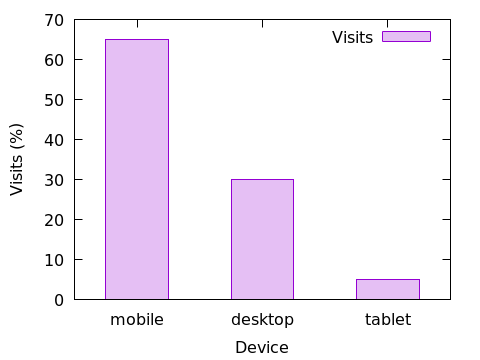### MXNet::NDArray

`MXNet::NDArray` is an indexable, multi-dimensional array that efficiently supports numerical operations (transposition, matrix multiplication, etc.).

All appropriate methods/commands accept instances of `MXNet::NDArray` with the correct shape (1-dimensional vectors for x-values, for example).

``````require "ishi"
require "mxnet"

m = MXNet::NDArray.array(
[[0, 0, 1, 1],
[1, 2, 3, 4],
[1, 1, 2, 2]]
)

figure = Ishi.new
charts = figure.charts(1, 2)
charts.plot(m, m, m)
charts.plot(m[.., 0], m[.., 1], m[.., 2])
figure.show
``````

### Extensions

By default, Ishi pops open a window to display charts. However, Ishi comes with extensions that display charts as text, HTML or inline images in the terminal; or that render charts to other `IO` destinations. Note: inline image display only works with ITerm2.

To plot the sin(x) function as text:

``````require "ishi/text" # or "ishi/html" or "ishi/iterm2"

Ishi.new do
plot("sin(x)")
end
``````

This produces:

``````    1 +--------------------------------------------------------------------+
|                *  *              +  *  **         +       *  *     |
0.8 |-+             *   *                 *    *          sin(x* *******-|
|              *     *                *    *               *    *    |
0.6 |-+            *      *              *     *               *     * +-|
|              *      *             *       *             *       *  |
0.4 |*+            *      *             *       *             *       *+-|
|*            *        *            *        *           *        *  |
0.2 |*+           *        *            *        *           *        *+-|
| *          *          *          *         *          *          * |
0 |-*          *          *          *         *          *          *-|
|  *         *          *         *           *         *           *|
-0.2 |-+*         *          *         *           *         *          +*|
|  *        *            *       *             *       *            *|
-0.4 |-+*        *            *       *             *       *           +*|
|   *      *              *      *             *      *              |
-0.6 |-+ *     *               *     *              *      *            +-|
|    *    *               *     *               *     *              |
-0.8 |-+   *   *                *   *                 *   *             +-|
|     *  *       +         **  *   +             *  *                |
-1 +--------------------------------------------------------------------+
-10              -5                 0                5                10
``````

## Contributors

```ishi:
github: toddsundsted/ishi
version: ~> 0.7.2```
Crystal 0.34.0

### Development Dependencies 2

• iterm2
`{'github' => 'toddsundsted/iterm2'}`
• spectator
`{'gitlab' => 'arctic-fox/spectator'}`

### Releases

Show all 9 releases
Last synced .